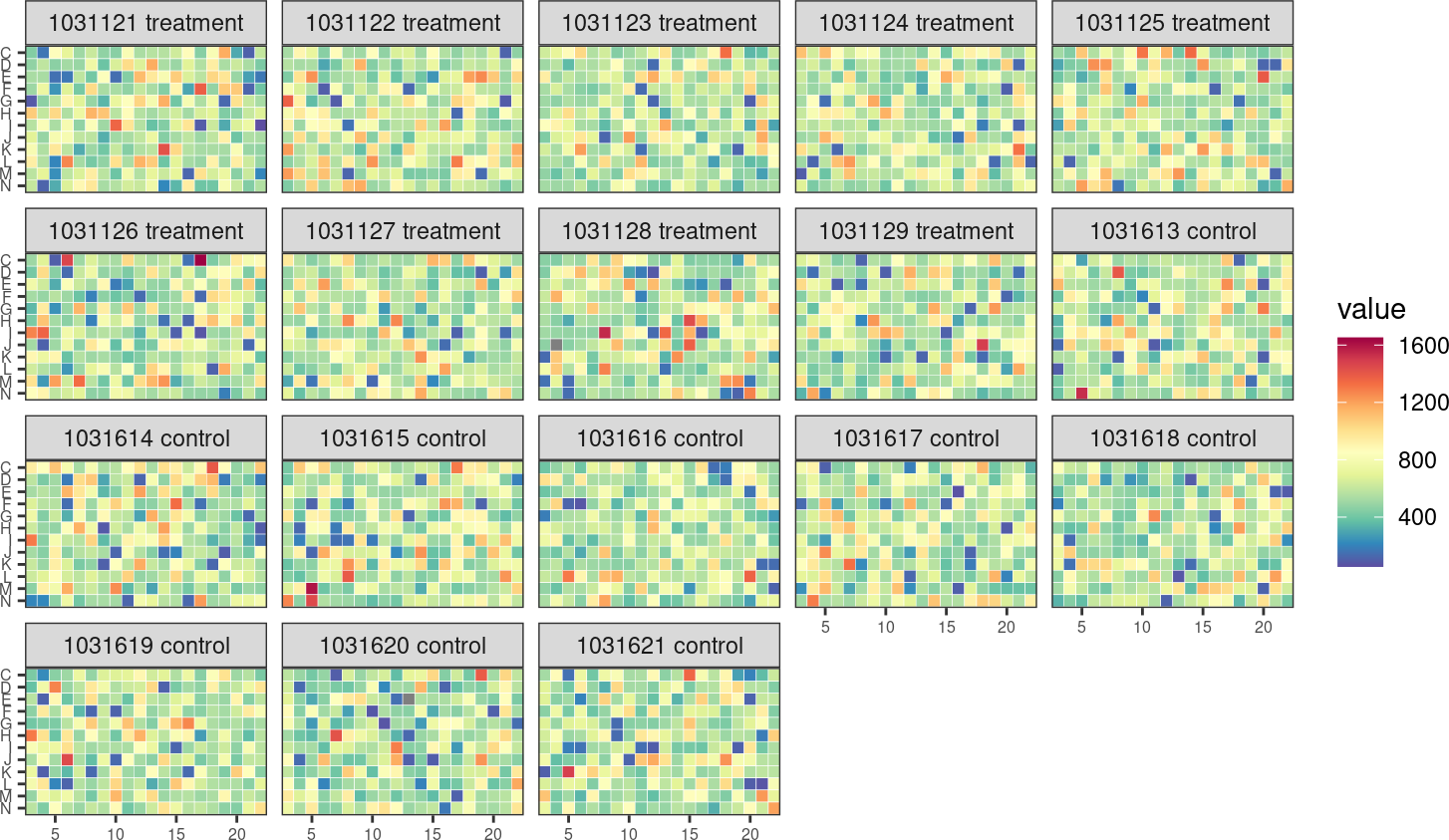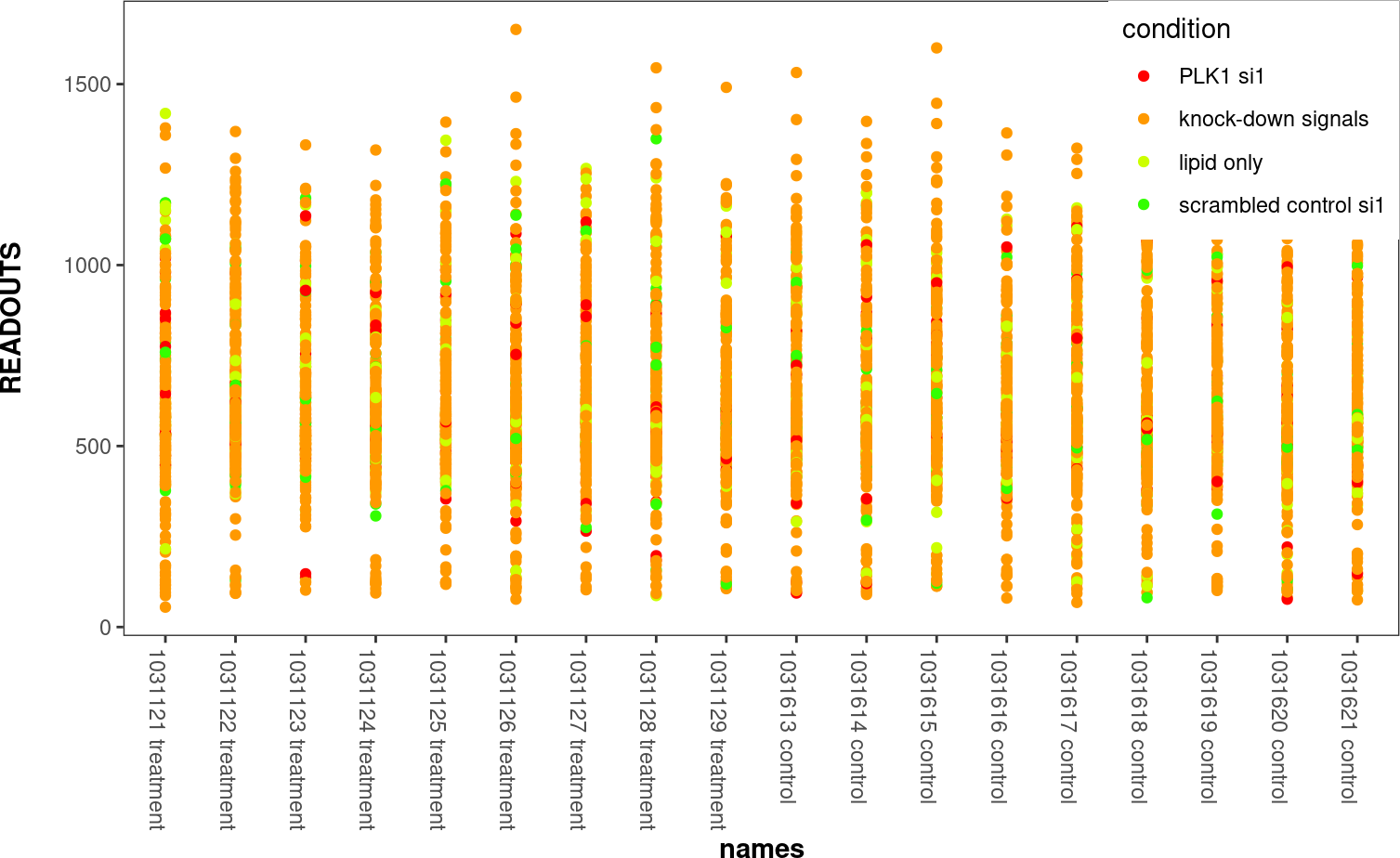# Contents

Package: sylsnet
Authors: Chunxuan Shao
Compiled: 2022-11-01

# 1 Introduction

Here is a step-by-step tutorial of the package synlet. This package processes data from cellBasedArrays. In this vignette, we will show a quick tour of using the synlet with dummy/stimulated data, including quality control, data visualization, and most importantly, hits selection.

# 2 Load the package and data.

First, Let’s have a look at the example data.

library(synlet)
## Loading required package: ggplot2
data("exampleDat")
head(exampleDat)
##     PLATE MASTER_PLATE     WELL_CONTENT_NAME  EXPERIMENT_TYPE
## 1 1031121         P001              AAK1 si3           sample
## 2 1031121         P001              PLK1 si1 control_positive
## 3 1031121         P001            lipid only control_negative
## 4 1031121         P001 scrambled control si1 control_negative
## 5 1031121         P001            lipid only control_negative
## 6 1031121         P001            AARSD1 si3           sample
## 1               treatment        C       10     455
## 2               treatment        C       11     814
## 3               treatment        C       12     537
## 4               treatment        C       13     568
## 5               treatment        C       14     566
## 6               treatment        C       15     632

The exampleDat is a data.frame containing 8 columns, all of them are mandatory and please do NOT change the column names. Besides that, users are free to add new columns. However, please not that the new columns may not appear in the final results. Users need to generate exampleDat like data.frame from the screen results.

In the exampleDat:

• PLATE, the name of individual plate. Must be numeric value.

• MASTER_PLATE, usually there are plate replicates designed in the RNAi screen experiments. Several individual PLATE having the identical layout are group to a MASTER_PLATE.

• WELL_CONTENT_NAME, the name of siRNA used in each cell, in the format of “geneName si(number)”, as usually several siRNAs are available to control for the off-target effect.

• EXPERIMENT_TYPE, indicates the cells are sample (interested knock-down), or negative control (control_negative), or positive control (control_positive).

• EXPERIMENT_MODIFICATION, there are two conditions in synthetic lethal RNAi screen, named treatment and control.

• ROW_NAME and COL_NAME, the position of siRNA reagent in the plate.

• READOUT, the output of RNAi screen output for each cell in the plate. Here the data are randomized.

The exampleDat contains three MASTER_PLATE, each MASTER_PLATE has three plates for treatment and control.

# 3 Quality control

## 3.1 Z and Z’ factor

Z’ factor and Z factor are widely used quality metrics in RNAi experiments.

Z’ factor = 1- 3*($$\delta_p$$ + $$\delta_n$$) / |$$\mu_p$$ - $$\mu_n$$|

Z factor = 1- 3*($$\delta_s$$ + $$\delta_n$$) / |$$\mu_s$$ - $$\mu_n$$|

In Z’ factor calculation, $$\delta_p$$, $$\delta_n$$, $$\mu_p$$, $$\mu_n$$ are the standard deviation and mean of positive control and negative control signal, respectively; while in Z factor formular $$\delta_s$$, $$\delta_n$$, $$\mu_s$$, $$\mu_n$$ are standard deviation and mean of samples and negative control signal, respectively. From the definition, we could see that Z’ factor measures the quality of optimization of plates, and Z factor accesses the performance of screen on actual samples. Generally, the plates with value >= 0.5 are considered as excellent assay. See  and  for more information and discussion.

By default $$\mu$$ in the denominator are mean value of signals, zFactor function offers the option to use median instead, which could be more robust in certain conditions.

Here is an example, we specify negative control is “scrambled control si1”, and positive control is “PLK1 si1”. These informations are stored in EXPERIMENT_TYPE column.

res <- zFactor(exampleDat, negativeCon = "scrambled control si1", positiveCon = "PLK1 si1")
## --- number of plates to calculate Z factor: 18---
head(res)
##            zFactor zPrimeFactor
## 1031121  -10.63422    -41.36317
## 1031122  -33.61932   -605.06754
## 1031123  -23.36895    -30.10814
## 1031124  -32.31389    -14.53771
## 1031125 -115.06929   -137.87454
## 1031126  -23.08055    -51.94328

As the READOUT are shuffled data, not surprising the Z and Z’ factor are negative value.

## 3.2 Heatmap of screen data

Synlet could plot the screen results in the format of heatmap, in which a dot represents a single cell. All plates are organized together in single figure. This provides a general view of RNAi screen quality.

plateHeatmap(exampleDat)## 3.3 Scatter plot of screen data

Usually in each plate there are negative and positive control siRNAs, which set the bound of siRNA effect. The scatter plot provide a direct way to examine the bound of controls. It is possible to specify multiple positive / negative controls in the function scatterPlot, and the output of all plates are arranged in a single plot.

scatterPlot(exampleDat, controlOnly = FALSE, colour = rainbow(10), "PLK1 si1", "scrambled control si1", "lipid only")
## Warning: Removed 2 rows containing missing values (geom_point).## 3.4 Knock-down effect

It is often intuitive to look at the knock-down effect of a single gene in RNAi screen experiments. The function siRNAPlot plot the normalized and raw signals, the positive and negative control signals, and Z’ factor of plates into a single graph. siRNAPlot provides the option to specify control siRNAs and normalization methods. The following codes show the knock-down effect of “AAK1”.

We need to calculate the Z’ factor based on mean and median of signals. By default, a pdf file named “AAK1.pdf” will be generated in the working directory, the return value contains all subplot and could be plotted separately.

zF_mean <- zFactor(exampleDat, negativeCon = "scrambled control si1", positiveCon = "PLK1 si1")
## --- number of plates to calculate Z factor: 18---
zF_med <- zFactor(exampleDat, negativeCon = "scrambled control si1", positiveCon = "PLK1 si1", useMean = FALSE)
## --- number of plates to calculate Z factor: 18---
tem.plot <- siRNAPlot("AAK1", exampleDat, controlsiRNA = c("lipid only", "scrambled control si1"), FILEPATH = ".",  zPrimeMed = zF_med,
zPrimeMean = zF_mean, treatment = "treatment", control = "control", normMethod = c("PLATE", "lipid only", "scrambled control si1"))
## +++ ProcessingAAK1+++
## Using siRNA, norMethod as id variables